## 4.2.4 Specific Heat

The lattice specific heat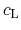is modeled by the following expression proposed in :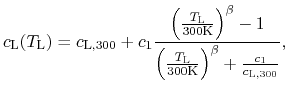where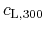is the value of the specific heat at 300 K and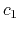and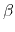are fitting parameters. Fig. 4.6 shows a comparison between the calibrated model (values are listed in Table 4.5) and measurements as a function of temperature for GaN. The results both of Kremer et al.  and Danilchenko et al.  are from bulk wurtzite GaN while most previous measurements were conducted on polycrystalline powder samples. Our model is calibrated against the model of Danilchenko et al., which is in excellent agreement with their own measurements for the heat capacity in the 0 K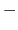300 K range and also with experimental results given in .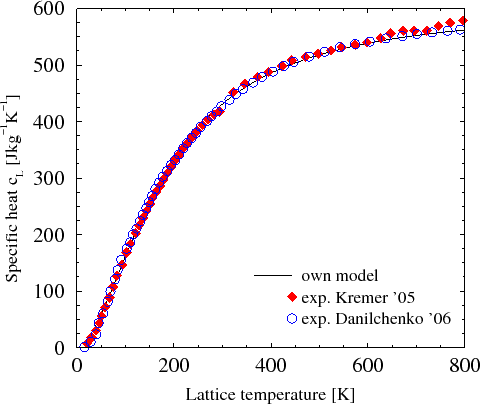Material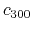[J/ kg K] [J/ kg K] GaN 431 171 1.75 AlN 748 482 2.29 InN 296 108 1.5

The parameter values for AlN are based on the summarized results in .

For the heat capacity of InN several works are based on the experimental data provided by Krukowski et al. . Their results are shown in Fig. 4.7 together with the models of Leitner et al.  and Zieborak et al. , which both provide models based on measurement results. In the present work the model is calibrated against the data in .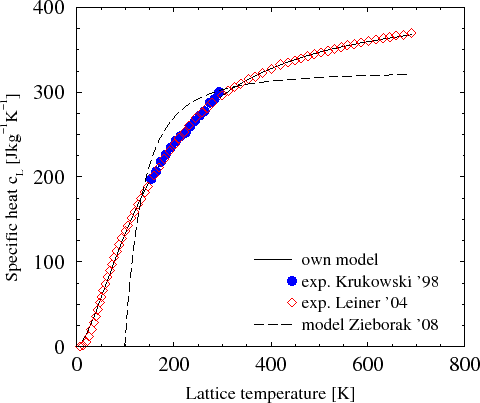The specific heat coefficients for alloy materials are expressed by a linear interpolation between the values of the binary materials :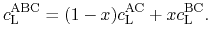S. Vitanov: Simulation of High Electron Mobility Transistors